Deutsche VersionConversion
Factor, Ratio, or Gain to a Level Value
(Gain Decibels dB) and vice versa

 The decibel is an auxiliary unit that indicates the ratio of two field quantities (voltage) or energy quantities (power). When using a fixed selected reference value, it may also be used as a pseudo unit (level). The decibel (dB) is a means of expressing the gain of an active device (such as an amplifier) or the loss in a passive device (such as an attenuator or the length of a cable). It is simply the ratio of the voltage at the output to the voltage at the input, expressed in logarithmic form. The technical term for an amplifier's output/input magnitude ratio is gain. As a ratio of equal units, like voltage out / voltage in, gain is naturally a unitless measurement. Mathematically, gain is symbolized by the capital letter "A".

Distinguish the field quantities (amplitude), such as voltage V or sound pressure p as sound field quantity and the
energy quantities, (or power quantities), such as power P or sound intensity I as sound energy quantity.

There is the factor (field quantity, amplitude) = ratio x/x0 and the factor (energy quantity) = ratio y/y0.
Electric power P ~ V2 (voltage squared) or sound intensity I ~ p2 (sound pressure squared).

 In audio, gain = amplification factor is a measure of the ability of the amplifier to increase the amplitude (or very rarely the power) of a signal from the input to the output. It is the amplification usually defined as the mean ratio of the signal output of a system to the signal input of the same system. It may also be defined on a logarithmic scale, in terms of the decimal logarithm of the same ratio ("dB gain"). A gain greater than one (zero dB), that is amplification, is the defining property of an active circuit, while a passive circuit will have a gain of less than one, called damping loss; see: Calculation: amplification (gain) and damping (loss) as level in decibels (dB) The decibels of gain should never need a suffix.

Conversion: Factor (Ratio) to Gain (Decibels)
and Gain (Decibels) to a Factor (Ratio)

 Simply enter the value to the left or the right side. The calculator works in both directions of the ↔ sign.

 ● Factor x (field quantity) ↔ ● Level Lx (voltage, sound pressure) dB (amplitude) gain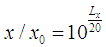Reference factor (field quantity) x0 = 1 ≡ 0 dB (amplitude)

Field quantity x always use the RMS value - but don't do that for the energy quantity y.

 Factor y (energy quantity) ↔ Level Ly (power, sound intensity)  dB (energy) gain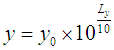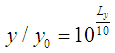Reference factor (energy quantity) y0 = 1 ≡ 0 dB (power)

Energy quantity y is field quantityx (amplitude rms) squared: y ~ x2.

 Electrical values Acoustical values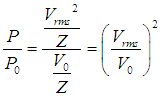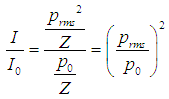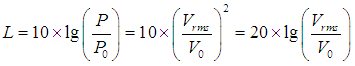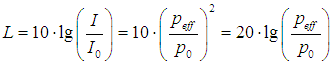Ratios and levels in electrical engineering and acoustics

Conversion: Gain G, Voltage ratio AV, and Power ratio AP
 Gain G dB | Voltage ratio (factor) AV | Power ratio (factor) AP | | ↓ | ↓ | ↓ | | Voltage ratio (factor) AV | Voltage (amplitude) gain GV dB | Power (energy) gain GP dB Power ratio (factor) AP | |
 Voltage gain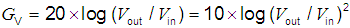in dB Power gain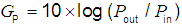in dB Voltage ratio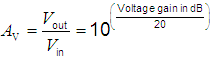= amplification factor (voltage) Power ratio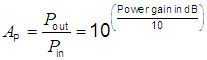= amplification factor (power)V1 = Vin and V2 = Vout. V2 > V1 or Vout > Vin means amplification. The dB value is positive. (+) V2 < V1 or Vout < Vin means damping. The dB value is negative. (−) V2 / V1 or Vout / Vin means the ratio. The amplification or the damping in dB is: L = 20 × log (voltage ratio V2 / V1) in dB.        V1 = Vin is the reference.

Conversion of voltage to voltage level

Conversion of sound units SPL (levels)

Common Gains and Losses Expressed in Decibels
Notice: Power gain is not used in audio engineering.

 Gain/loss      as a ratio (out/in) Gain/loss      as a factor Gain/loss in dB     Field quantities   (Voltage gain) Gain/loss in dB   Energy quantities (Power gain) 10,000:1 10,000 +80.00 dB +40.00 dB 1,000:1 1,000 +60.00 dB +30.00 dB 100:1 100 +40.00 dB +20.00 dB 10:1 10 +20.00 dB +10.00 dB 5:1 5 +13.98 dB +6.99 dB 4:1 4 +12.04 dB +6.02 dB ● 2:1 2 +6.02 dB ● +3.01 dB 1:1 1 0,00 dB 0.00 dB 1:2 0.5 −6.02 dB ● −3.01 dB 1:4 0.25 −12.04 dB −6.02 dB ● 1:5 0.2 −13.98 dB −6.99 dB 1:10 0.1 −20.00 dB −10.00 dB 1:100 0.01 −40.00 dB −20.00 dB 1:1,000 0.001 −60.00 dB −30.00 dB 1:10,000 0.0001 −80.00 dB −40.00 dB

40 dB voltage gain (amplitude) is 100 times the voltage factor (amplitude).
40 dB power gain is 10,000 times the power factor (energy).

Voltage Gain and Power Gain

What is Amplitude? − Amplitude of a sound

 Voltage gain (amplitude) Power gain (energy) Voltage ratio Factor V2/V1 Voltage amplification GV in dB Power ratio Factor P2/P1 Power amplification GP in dB 103 +60 106 +60 102 +40 104 +40 101 +20 102 +20 √10 = 3.16 +10 10 +10 2 +6 4 +6 √2 = 1.414 +3 2 +3 1 ±0 1 ±0 1/√2 = 0.7071 −3 1/2 = 0.5 −3 1/2 = 0.5 −6 1/4 = 0.25 −6 1/√10 = 0.316 −10 10−1 = 0.1 −10 10−1 = 0.1 −20 10−2 = 0.01 −20 10−2 = 0.01 −40 10−4 = 0.0001 −40 10−3 = 0.001 −60 10−6 = 0.000001 −60 V2/V1 = 10(GV in dB/20) GV = 20×log (V2/V1) P2/P1 = 10(GP in dB/10) GP = 10×log (P2/P1)

 In audio engineering the term "power gain" is really not used.   Loudness is English gain, level or volume. For amateurs, "gain" means the raising of the level of the "preamp" with a potentiometer, which is responsible for the distortion of the guitar signal of the "amp". The amplification factor, called gain, is the extent to which an analog amplifier boosts the strength of a signal. In an electronic amplifier, the input "signal" is usually a voltage. Gain in dB is the logarithmic ratio between the output and input voltage of the preamplifier. Typical values lie between 0 and 70 dB. Gains and losses may be expressed in terms of a unitless ratio, or in the unit of decibels (dB). A gain of factor 1 - equivalent to 0 dB - where both input and output are at the same voltage level is also known as "unity gain"

Factor, Power Gain and Voltage Gain

 The level of the output power level is 0 dB, that is 100% (factor or ratio = 1). The level of −3 dB is equivalent to 50% (factor = 0.5) and the level of −6 dB is equivalent to 25% (factor = 1/4 = 0.25) of the initial power. This applies to the energy quantity.   The level of the output voltage level is 0 dB, that is 100% (factor or ratio = 1). The level of −3 dB is equivalent to 70.7% (factor = 0.7071), and the level of −6 dB is equivalent to 50% (factor = 1/2 = 0.5) of the initial voltage. This applies to the field quantity.

 Acousticians and sound protectors ("noise fighters") need the sound intensity (acoustic intensity) – but sound engineers and sound designers ("ear people") don't need that sound energy quantity.   Who is involved in audio engineering, should rather take care of the sound field quantity, that is the sound pressure or the sound pressure level (SPL) as an effect at the eardrums of our hearing and on the membranes of the microphones, and the corresponding audio voltage and its voltage level.

 Such a logarithmic quantity is useful because e.g. the decibel gain values of several amplifiers in a sequence can simply be added to obtain the total gain of the amplifier chain. The number of decibels is 20 times the logarithm (to base 10) of the amplitude factor (ratio) as RMS value of a field quantity or alternatively 10 times the logarithm (to base 10) of the power amplification factor or loss factor.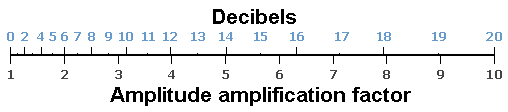Level L in decibel (dB) = 20 × log (x)       x = factor (amplitude, field quantity)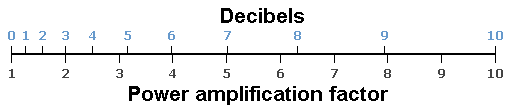Level L in decibel (dB) = 10 × log (y)       y = factor (power, energy quantity)

 A typical question: "16 dB how many times?" Answer: Do they mean a field quantity, like a sound pressure or an energy quantity, like a sound intensity? That is why the calculator gives two answers: The factor is: 6.31 or the factor is: 39.8 depending on field quantity or energy quantity. 6.312 (field quantity squared) = 39.8 (energy quantity).
 Gain Decibels (dB) Voltage factor field quantity Power factor energy quantity
 Questions: For an amplifier with an amplification factor of 100, calculate the following: a) voltage gain in dB. b) voltage gain at the cutoff frequency (break frequency) in dB. c) amplification factor at the cutoff frequency (break frequency). Answers: a) amplification factor 100 ≡ gain 40 dB b) gain at the cutoff frequency is 3 dB less − so it is 37 dB. c) gain 37 dB ≡ amplification factor 70.7 at the cutoff frequency.